# RS Aggarwal Solutions for Class 7 Maths Chapter 11 Profit and Loss

RS Aggarwal Solutions for Class 7 Maths Chapter 11 – Profit and Loss are available here, so that students can check for the solutions whenever they are facing difficulty while solving the questions from RS Aggarwal Solutions for Class 7. These solutions for Chapter 11 are available in PDF format so that students can download it and learn offline as well. This book is one of the top materials when it comes to providing a question bank to practice from.

We at BYJU’S have prepared the RS Aggarwal Solutions for Class 7 Maths Chapter 11 wherein, problems are formulated by our expert tutors to assist you with your exam preparation to attain good marks in Maths. Download pdf of Class 7 Chapter 11 in their respective links.

## Download PDF of RS Aggarwal Solutions for Class 7 Maths Chapter 11 – Profit and Loss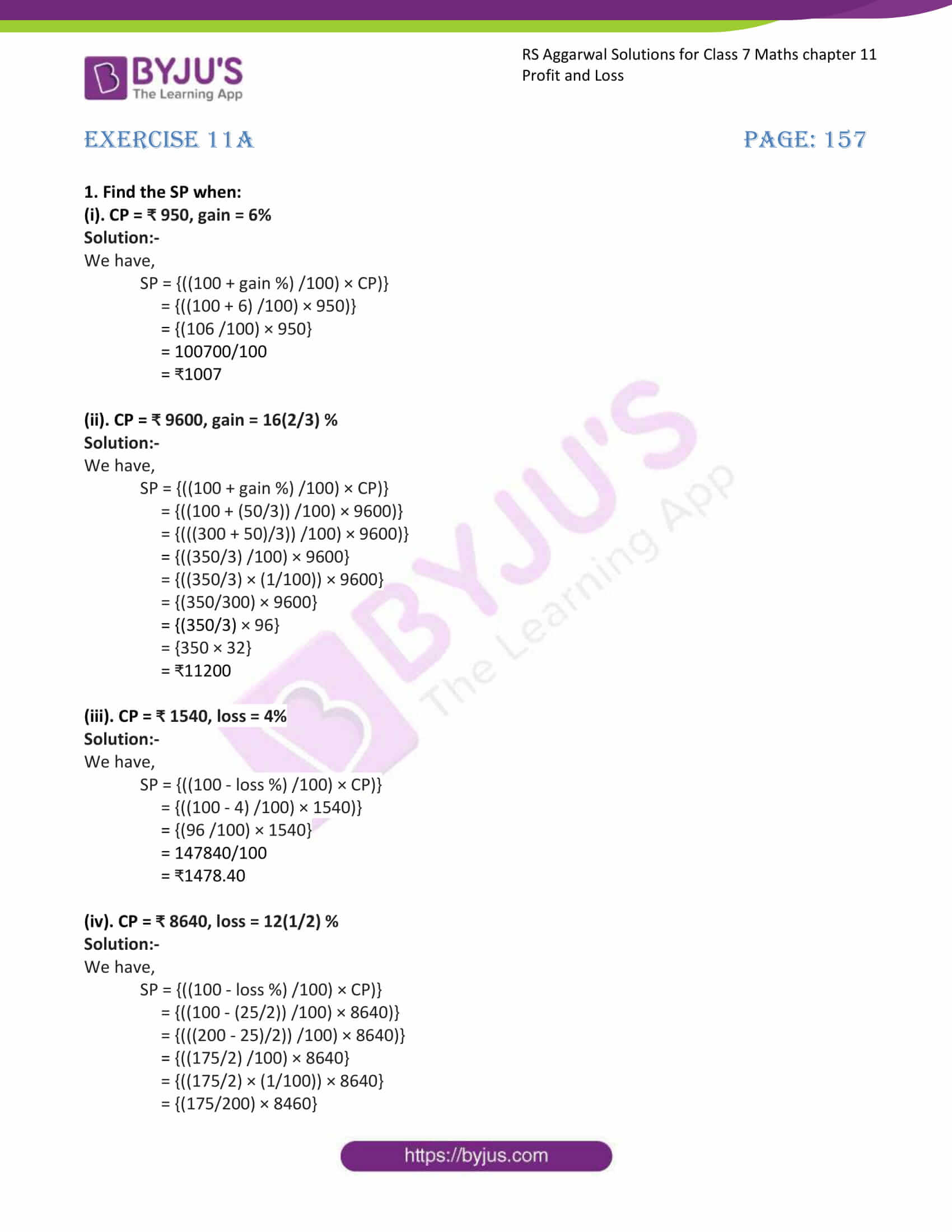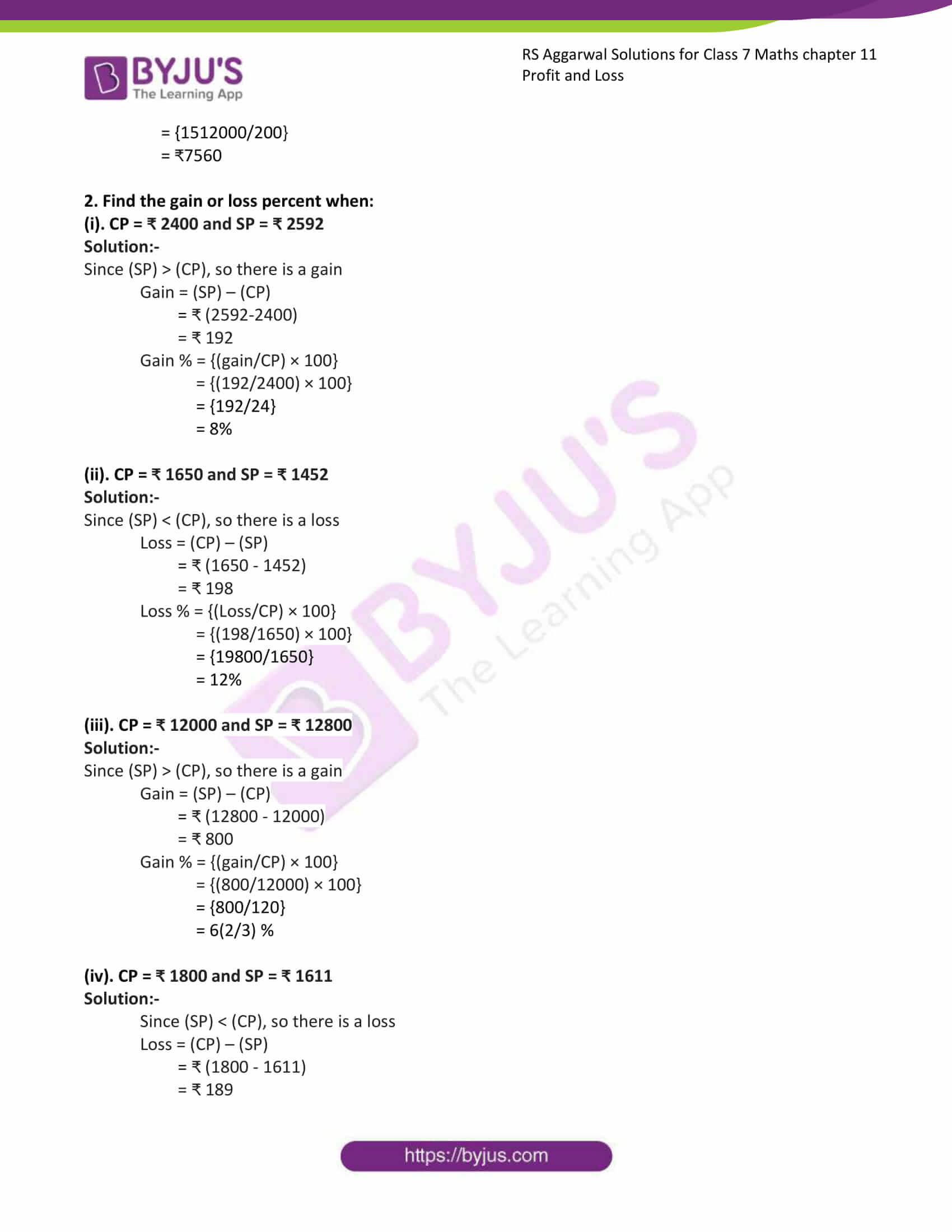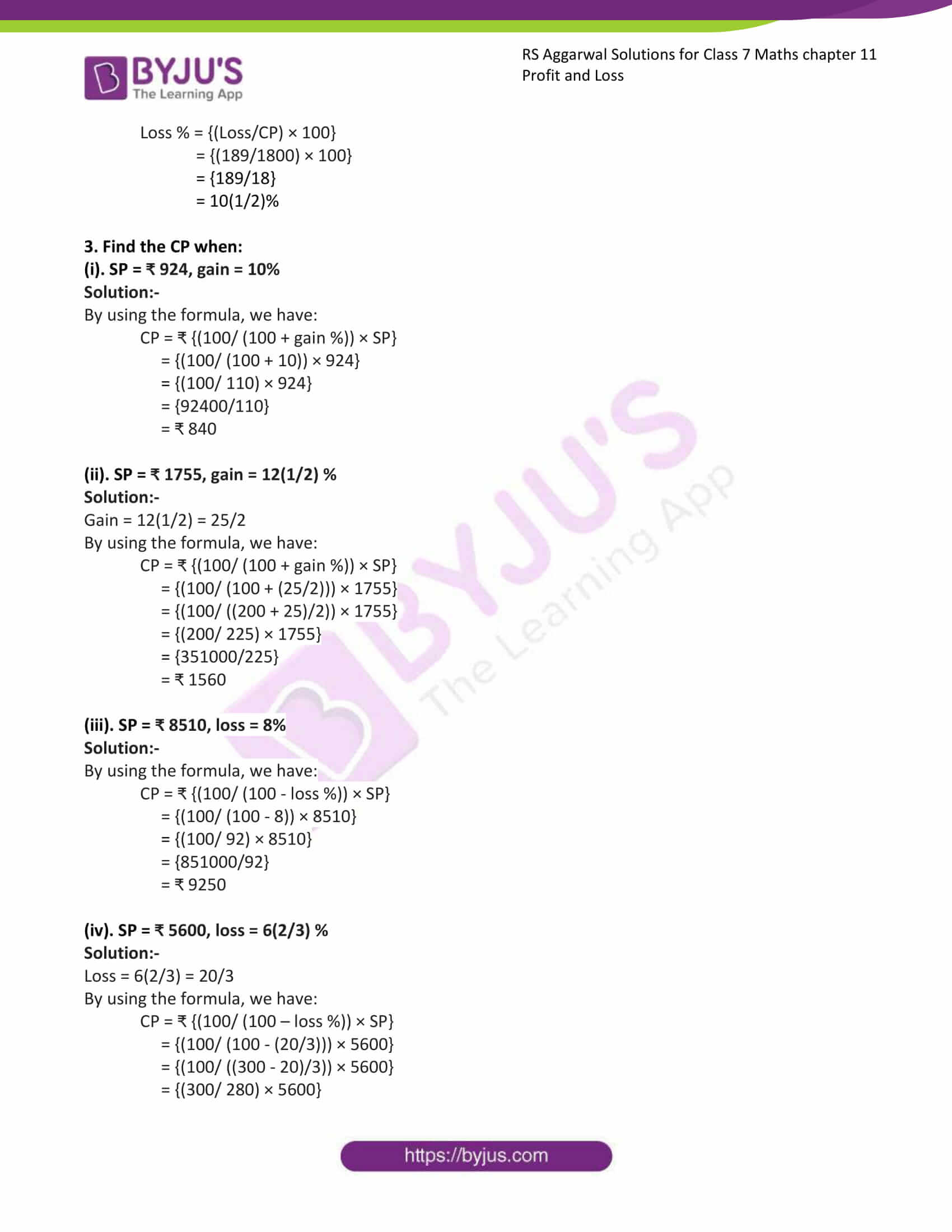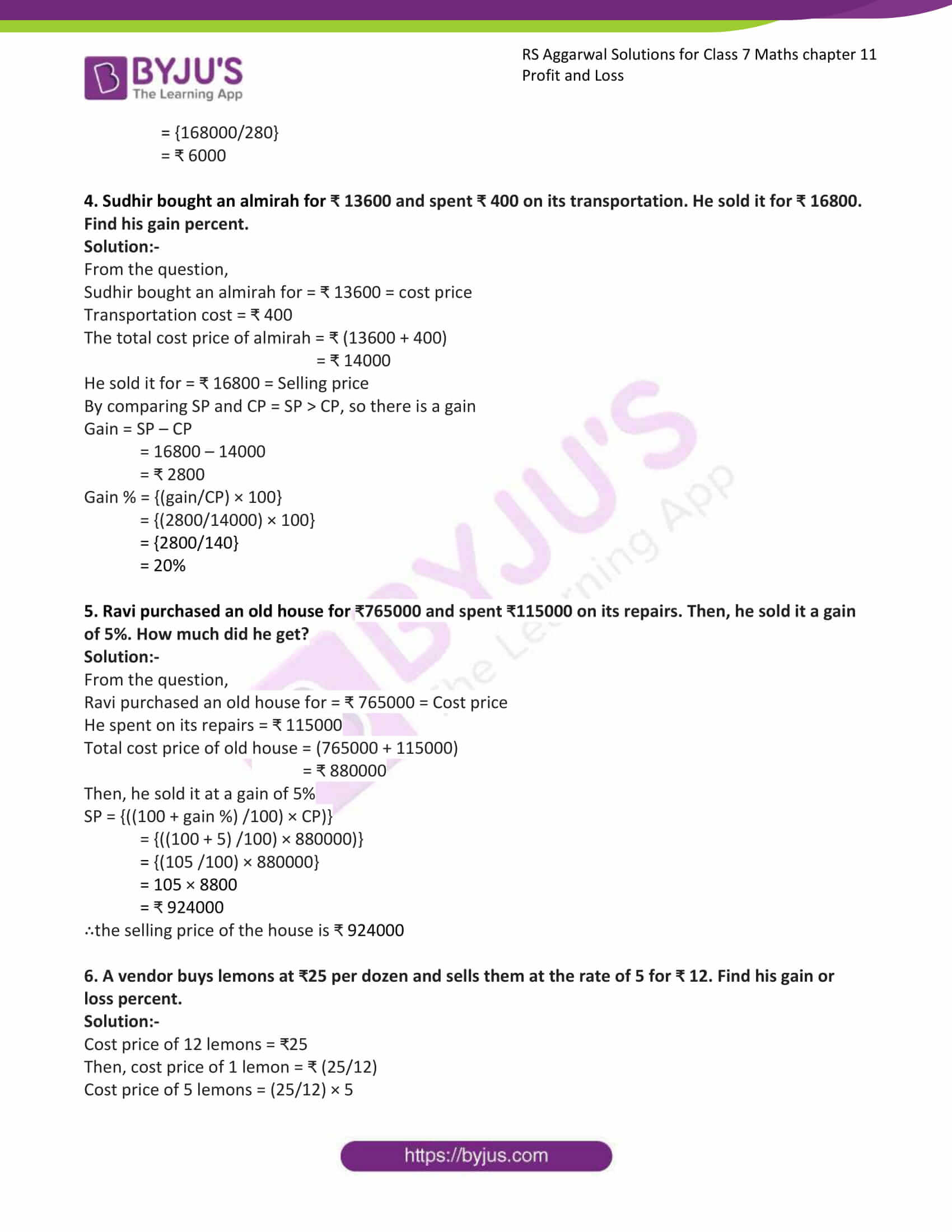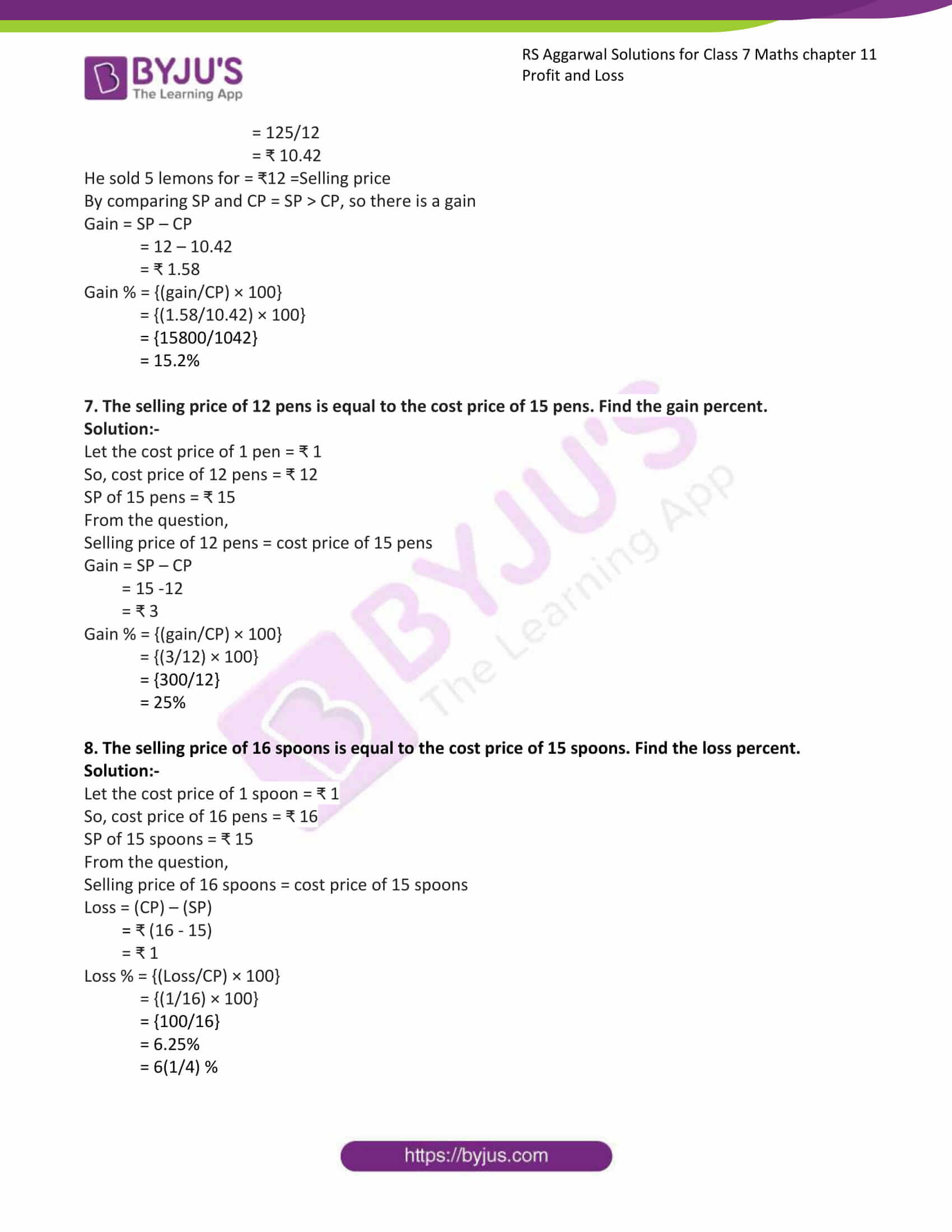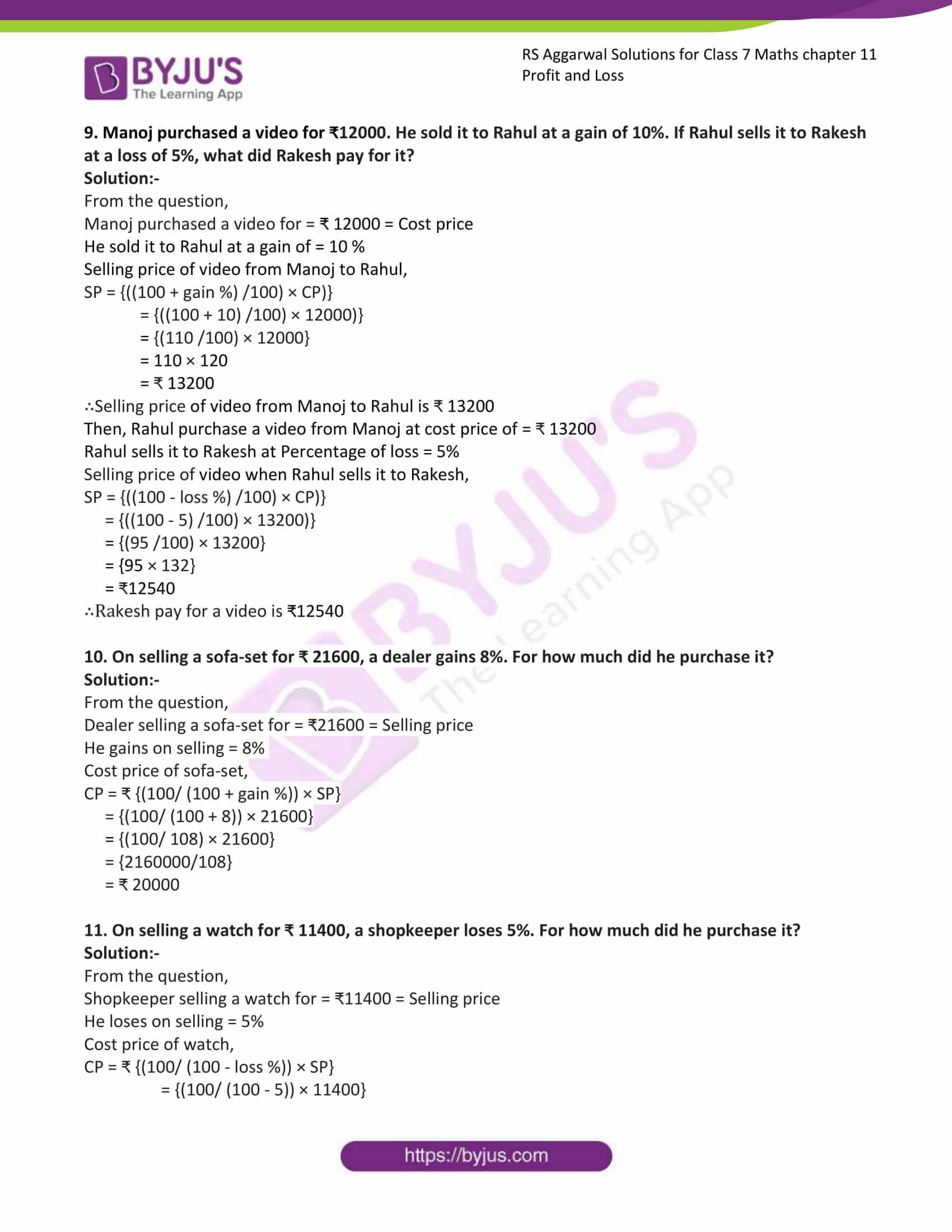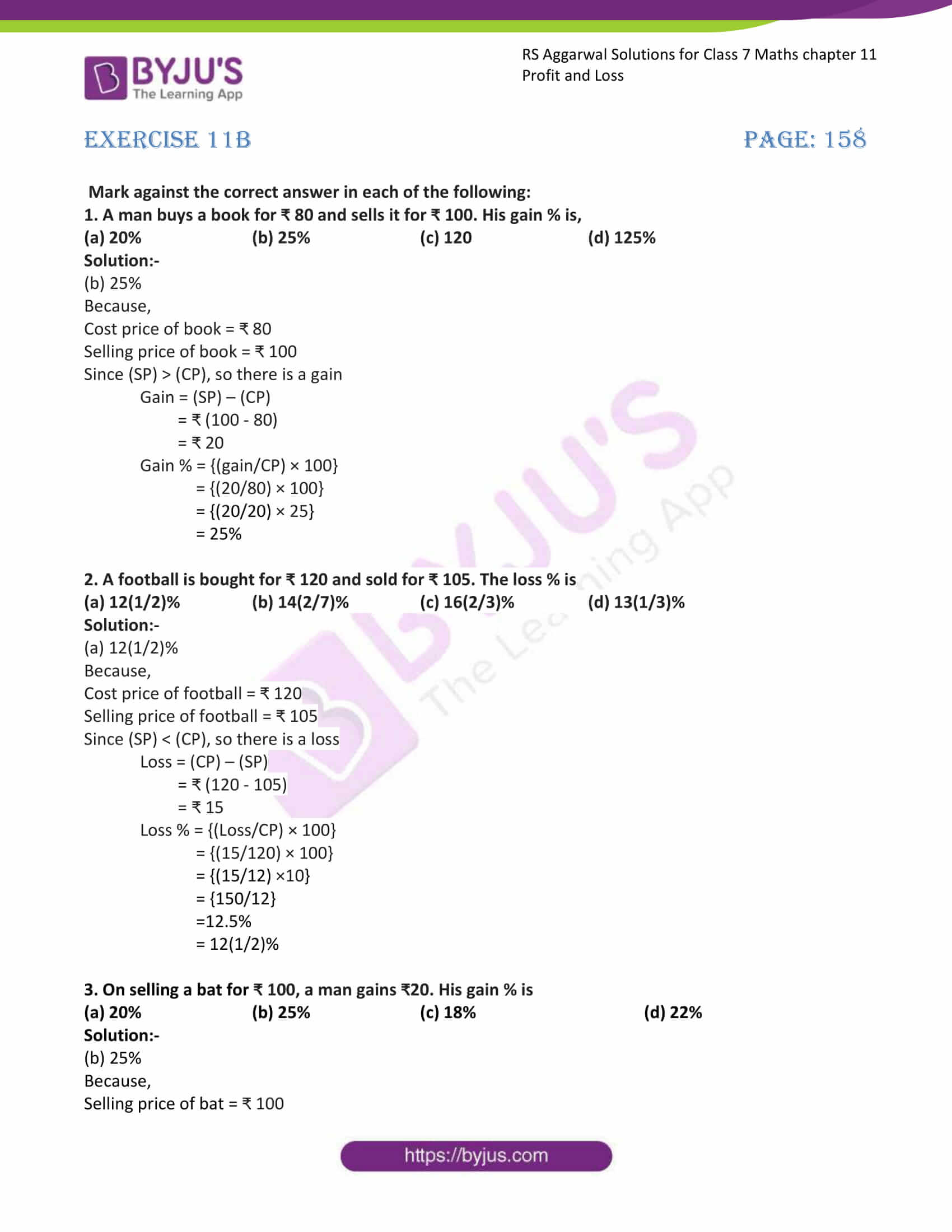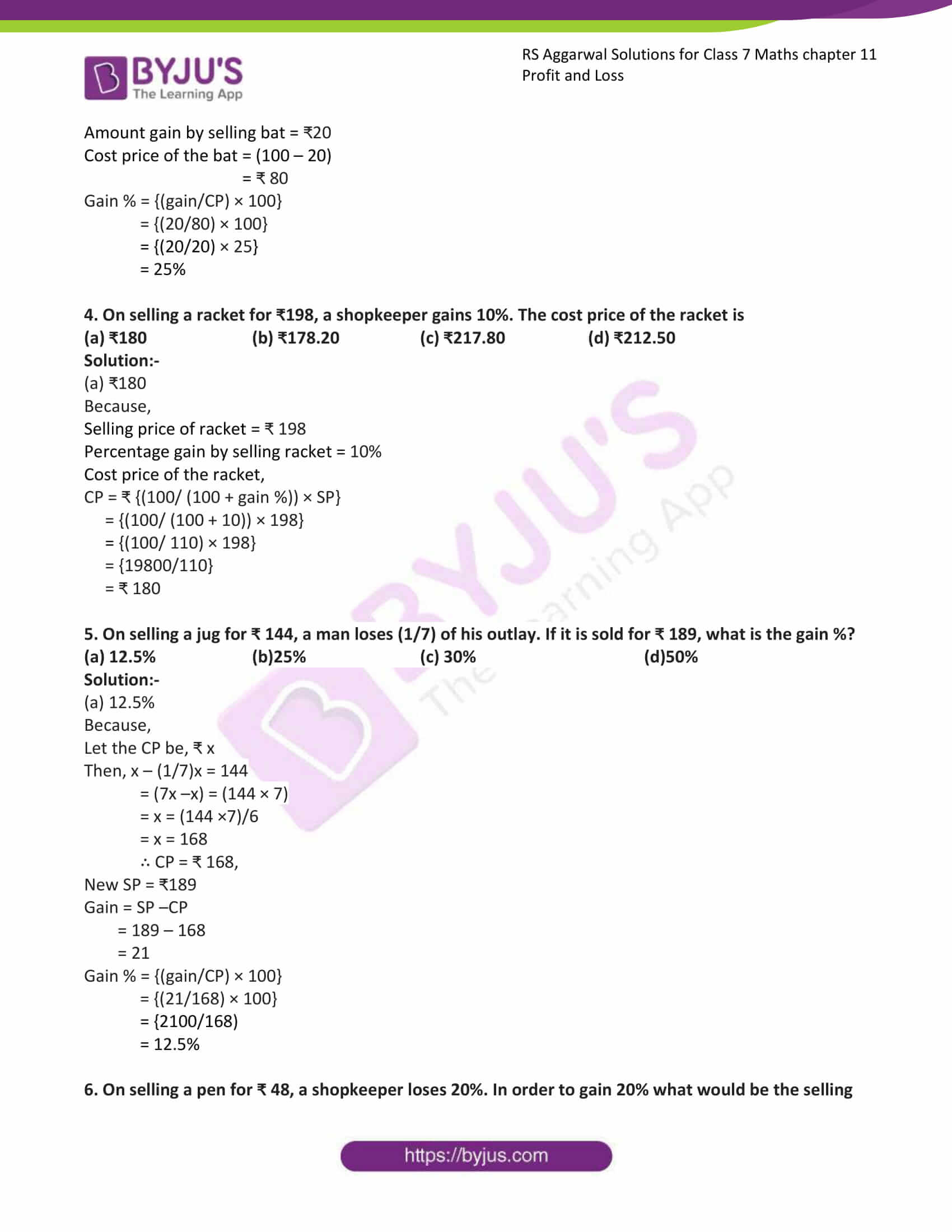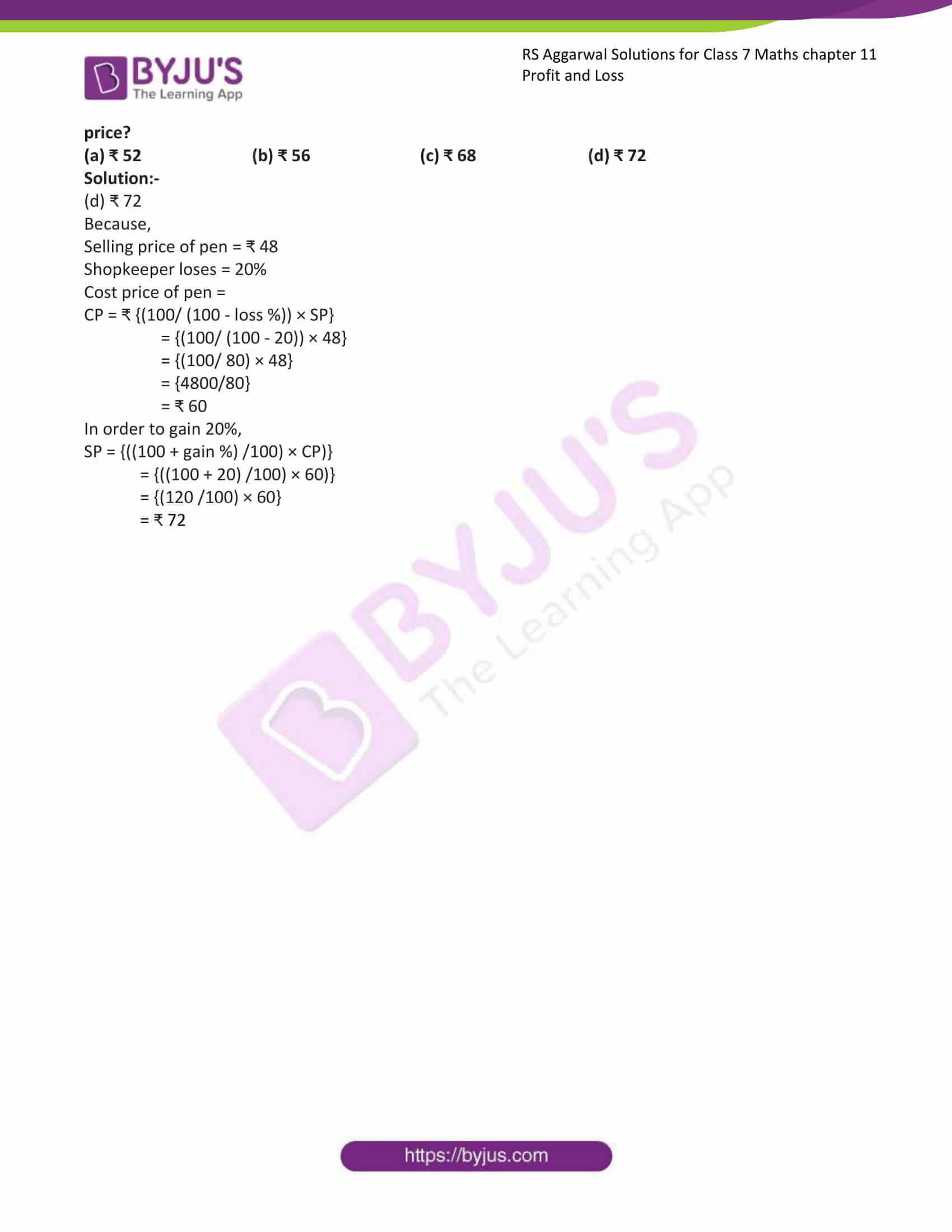### Also, access RS Aggarwal Solutions for Class 7 Chapter 11 Exercises

Exercise 11A

Exercise 11B

Exercise 11A

1. Find the SP when:

(i). CP = ₹ 950, gain = 6%

Solution:-

We have,

SP = {((100 + gain %) /100) × CP)}

= {((100 + 6) /100) × 950)}

= {(106 /100) × 950}

= 100700/100

= ₹1007

(ii). CP = ₹ 9600, gain = 16(2/3) %

Solution:-

We have,

SP = {((100 + gain %) /100) × CP)}

= {((100 + (50/3)) /100) × 9600)}

= {(((300 + 50)/3)) /100) × 9600)}

= {((350/3) /100) × 9600}

= {((350/3) × (1/100)) × 9600}

= {(350/300) × 9600}

= {(350/3) × 96}

= {350 × 32}

= ₹11200

(iii). CP = ₹ 1540, loss = 4%

Solution:-

We have,

SP = {((100 – loss %) /100) × CP)}

= {((100 – 4) /100) × 1540)}

= {(96 /100) × 1540}

= 147840/100

= ₹1478.40

(iv). CP = ₹ 8640, loss = 12(1/2) %

Solution:-

We have,

SP = {((100 – loss %) /100) × CP)}

= {((100 – (25/2)) /100) × 8640)}

= {(((200 – 25)/2)) /100) × 8640)}

= {((175/2) /100) × 8640}

= {((175/2) × (1/100)) × 8640}

= {(175/200) × 8460}

= {1512000/200}

= ₹7560

2. Find the gain or loss percent when:

(i). CP = ₹ 2400 and SP = ₹ 2592

Solution:-

Since (SP) > (CP), so there is a gain

Gain = (SP) – (CP)

= ₹ (2592-2400)

= ₹ 192

Gain % = {(gain/CP) × 100}

= {(192/2400) × 100}

= {192/24}

= 8%

(ii). CP = ₹ 1650 and SP = ₹ 1452

Solution:-

Since (SP) < (CP), so there is a loss

Loss = (CP) – (SP)

= ₹ (1650 – 1452)

= ₹ 198

Loss % = {(Loss/CP) × 100}

= {(198/1650) × 100}

= {19800/1650}

= 12%

(iii). CP = ₹ 12000 and SP = ₹ 12800

Solution:-

Since (SP) > (CP), so there is a gain

Gain = (SP) – (CP)

= ₹ (12800 – 12000)

= ₹ 800

Gain % = {(gain/CP) × 100}

= {(800/12000) × 100}

= {800/120}

= 6(2/3) %

(iv). CP = ₹ 1800 and SP = ₹ 1611

Solution:-

Since (SP) < (CP), so there is a loss

Loss = (CP) – (SP)

= ₹ (1800 – 1611)

= ₹ 189

Loss % = {(Loss/CP) × 100}

= {(189/1800) × 100}

= {189/18}

= 10(1/2)%

3. Find the CP when:

(i). SP = ₹ 924, gain = 10%

Solution:-

By using the formula, we have:

CP = ₹ {(100/ (100 + gain %)) × SP}

= {(100/ (100 + 10)) × 924}

= {(100/ 110) × 924}

= {92400/110}

= ₹ 840

(ii). SP = ₹ 1755, gain = 12(1/2) %

Solution:-

Gain = 12(1/2) = 25/2

By using the formula, we have:

CP = ₹ {(100/ (100 + gain %)) × SP}

= {(100/ (100 + (25/2))) × 1755}

= {(100/ ((200 + 25)/2)) × 1755}

= {(200/ 225) × 1755}

= {351000/225}

= ₹ 1560

(iii). SP = ₹ 8510, loss = 8%

Solution:-

By using the formula, we have:

CP = ₹ {(100/ (100 – loss %)) × SP}

= {(100/ (100 – 8)) × 8510}

= {(100/ 92) × 8510}

= {851000/92}

= ₹ 9250

(iv). SP = ₹ 5600, loss = 6(2/3) %

Solution:-

Loss = 6(2/3) = 20/3

By using the formula, we have:

CP = ₹ {(100/ (100 – loss %)) × SP}

= {(100/ (100 – (20/3))) × 5600}

= {(100/ ((300 – 20)/3)) × 5600}

= {(300/ 280) × 5600}

= {168000/280}

= ₹ 6000

4. Sudhir bought an almirah for ₹ 13600 and spent ₹ 400 on its transportation. He sold it for ₹ 16800. Find his gain percent.

Solution:-

From the question,

Sudhir bought an almirah for = ₹ 13600 = cost price

Transportation cost = ₹ 400

The total cost price of almirah = ₹ (13600 + 400)

= ₹ 14000

He sold it for = ₹ 16800 = Selling price

By comparing SP and CP = SP > CP, so there is a gain

Gain = SP – CP

= 16800 – 14000

= ₹ 2800

Gain % = {(gain/CP) × 100}

= {(2800/14000) × 100}

= {2800/140}

= 20%

5. Ravi purchased an old house for ₹765000 and spent ₹115000 on its repairs. Then, he sold it a gain of 5%. How much did he get?

Solution:-

From the question,

Ravi purchased an old house for = ₹ 765000 = Cost price

He spent on its repairs = ₹ 115000

Total cost price of old house = (765000 + 115000)

= ₹ 880000

Then, he sold it at a gain of 5%

SP = {((100 + gain %) /100) × CP)}

= {((100 + 5) /100) × 880000)}

= {(105 /100) × 880000}

= 105 × 8800

= ₹ 924000

∴the selling price of the house is ₹ 924000

6. A vendor buys lemons at ₹25 per dozen and sells them at the rate of 5 for ₹ 12. Find his gain or loss percent.

Solution:-

Cost price of 12 lemons = ₹25

Then, cost price of 1 lemon = ₹ (25/12)

Cost price of 5 lemons = (25/12) × 5

= 125/12

= ₹ 10.42

He sold 5 lemons for = ₹12 =Selling price

By comparing SP and CP = SP > CP, so there is a gain

Gain = SP – CP

= 12 – 10.42

= ₹ 1.58

Gain % = {(gain/CP) × 100}

= {(1.58/10.42) × 100}

= {15800/1042}

= 15.2%

7. The selling price of 12 pens is equal to the cost price of 15 pens. Find the gain percent.

Solution:-

Let the cost price of 1 pen = ₹ 1

So, cost price of 12 pens = ₹ 12

SP of 15 pens = ₹ 15

From the question,

Selling price of 12 pens = cost price of 15 pens

Gain = SP – CP

= 15 -12

= ₹ 3

Gain % = {(gain/CP) × 100}

= {(3/12) × 100}

= {300/12}

= 25%

8. The selling price of 16 spoons is equal to the cost price of 15 spoons. Find the loss percent.

Solution:-

Let the cost price of 1 spoon = ₹ 1

So, cost price of 16 pens = ₹ 16

SP of 15 spoons = ₹ 15

From the question,

Selling price of 16 spoons = cost price of 15 spoons

Loss = (CP) – (SP)

= ₹ (16 – 15)

= ₹ 1

Loss % = {(Loss/CP) × 100}

= {(1/16) × 100}

= {100/16}

= 6.25%

= 6(1/4) %

9. Manoj purchased a video for ₹12000. He sold it to Rahul at a gain of 10%. If Rahul sells it to Rakesh at a loss of 5%, what did Rakesh pay for it?

Solution:-

From the question,

Manoj purchased a video for = ₹ 12000 = Cost price

He sold it to Rahul at a gain of = 10 %

Selling price of video from Manoj to Rahul,

SP = {((100 + gain %) /100) × CP)}

= {((100 + 10) /100) × 12000)}

= {(110 /100) × 12000}

= 110 × 120

= ₹ 13200

∴Selling price of video from Manoj to Rahul is ₹ 13200

Then, Rahul purchase a video from Manoj at cost price of = ₹ 13200

Rahul sells it to Rakesh at Percentage of loss = 5%

Selling price of video when Rahul sells it to Rakesh,

SP = {((100 – loss %) /100) × CP)}

= {((100 – 5) /100) × 13200)}

= {(95 /100) × 13200}

= {95 × 132}

= ₹12540

∴Rakesh pay for a video is ₹12540

10. On selling a sofa-set for ₹ 21600, a dealer gains 8%. For how much did he purchase it?

Solution:-

From the question,

Dealer selling a sofa-set for = ₹21600 = Selling price

He gains on selling = 8%

Cost price of sofa-set,

CP = ₹ {(100/ (100 + gain %)) × SP}

= {(100/ (100 + 8)) × 21600}

= {(100/ 108) × 21600}

= {2160000/108}

= ₹ 20000

11. On selling a watch for ₹ 11400, a shopkeeper loses 5%. For how much did he purchase it?

Solution:-

From the question,

Shopkeeper selling a watch for = ₹11400 = Selling price

He loses on selling = 5%

Cost price of watch,

CP = ₹ {(100/ (100 – loss %)) × SP}

= {(100/ (100 – 5)) × 11400}

= {(100/ 95) × 11400}

= {11400} × 95

= ₹ 12000

Exercise 11B

Mark against the correct answer in each of the following:

1. A man buys a book for ₹ 80 and sells it for ₹ 100. His gain % is,

(a) 20% (b) 25% (c) 120 (d) 125%

Solution:-

(b) 25%

Because,

Cost price of book = ₹ 80

Selling price of book = ₹ 100

Since (SP) > (CP), so there is a gain

Gain = (SP) – (CP)

= ₹ (100 – 80)

= ₹ 20

Gain % = {(gain/CP) × 100}

= {(20/80) × 100}

= {(20/20) × 25}

= 25%

2. A football is bought for ₹ 120 and sold for ₹ 105. The loss % is

(a) 12(1/2)% (b) 14(2/7)% (c) 16(2/3)% (d) 13(1/3)%

Solution:-

(a) 12(1/2)%

Because,

Cost price of football = ₹ 120

Selling price of football = ₹ 105

Since (SP) < (CP), so there is a loss

Loss = (CP) – (SP)

= ₹ (120 – 105)

= ₹ 15

Loss % = {(Loss/CP) × 100}

= {(15/120) × 100}

= {(15/12) ×10}

= {150/12}

=12.5%

= 12(1/2)%

3. On selling a bat for ₹ 100, a man gains ₹20. His gain % is

(a) 20% (b) 25% (c) 18% (d) 22%

Solution:-

(b) 25%

Because,

Selling price of bat = ₹ 100

Amount gain by selling bat = ₹20

Cost price of the bat = (100 – 20)

= ₹ 80

Gain % = {(gain/CP) × 100}

= {(20/80) × 100}

= {(20/20) × 25}

= 25%

4. On selling a racket for ₹198, a shopkeeper gains 10%. The cost price of the racket is

(a) ₹180 (b) ₹178.20 (c) ₹217.80 (d) ₹212.50

Solution:-

(a) ₹180

Because,

Selling price of racket = ₹ 198

Percentage gain by selling racket = 10%

Cost price of the racket,

CP = ₹ {(100/ (100 + gain %)) × SP}

= {(100/ (100 + 10)) × 198}

= {(100/ 110) × 198}

= {19800/110}

= ₹ 180

5. On selling a jug for ₹ 144, a man loses (1/7) of his outlay. If it is sold for ₹ 189, what is the gain %?

(a) 12.5% (b)25% (c) 30% (d)50%

Solution:-

(a) 12.5%

Because,

Let the CP be, ₹ x

Then, x – (1/7)x = 144

= (7x –x) = (144 × 7)

= x = (144 ×7)/6

= x = 168

∴ CP = ₹ 168,

New SP = ₹189

Gain = SP –CP

= 189 – 168

= 21

Gain % = {(gain/CP) × 100}

= {(21/168) × 100}

= {2100/168)

= 12.5%

6. On selling a pen for ₹ 48, a shopkeeper loses 20%. In order to gain 20% what would be the selling price?

(a) ₹ 52 (b) ₹ 56 (c) ₹ 68 (d) ₹ 72

Solution:-

(d) ₹ 72

Because,

Selling price of pen = ₹ 48

Shopkeeper loses = 20%

Cost price of pen =

CP = ₹ {(100/ (100 – loss %)) × SP}

= {(100/ (100 – 20)) × 48}

= {(100/ 80) × 48}

= {4800/80}

= ₹ 60

In order to gain 20%,

SP = {((100 + gain %) /100) × CP)}

= {((100 + 20) /100) × 60)}

= {(120 /100) × 60}

= ₹ 72

## RS Aggarwal Solutions for Class 7 Maths Chapter 11 – Profit and Loss

Chapter 11 – Profit and Loss contains 2 exercises and the RS Aggarwal Solutions available on this page provide solutions to the questions present in the exercises. Now, let us have a look at some of the concepts discussed in this chapter.

• Some Terms Related to Profit and Loss
• To Find SO When CP and Gain% or Loss% are Given
• To Find CP when SP and Gain% or Loss% are Given

### Chapter Brief of RS Aggarwal Solutions for Class 7 Maths Chapter 11 – Profit and Loss

RS Aggarwal Solutions for Class 7 Maths Chapter 11 – Profit and Loss. The money paid by the shopkeeper to buy the products from wholesalers is called Cost Price. The rate at which the shopkeeper sells the products is called Selling Price. Profit and Loss are entirely based on cost price and selling price. In this chapter, students are going to learn the percentage gain and also percentage loss.